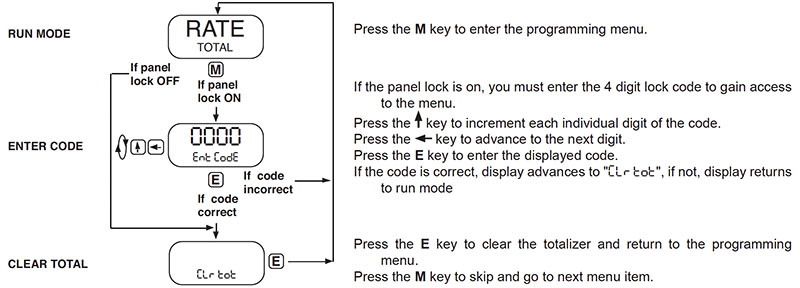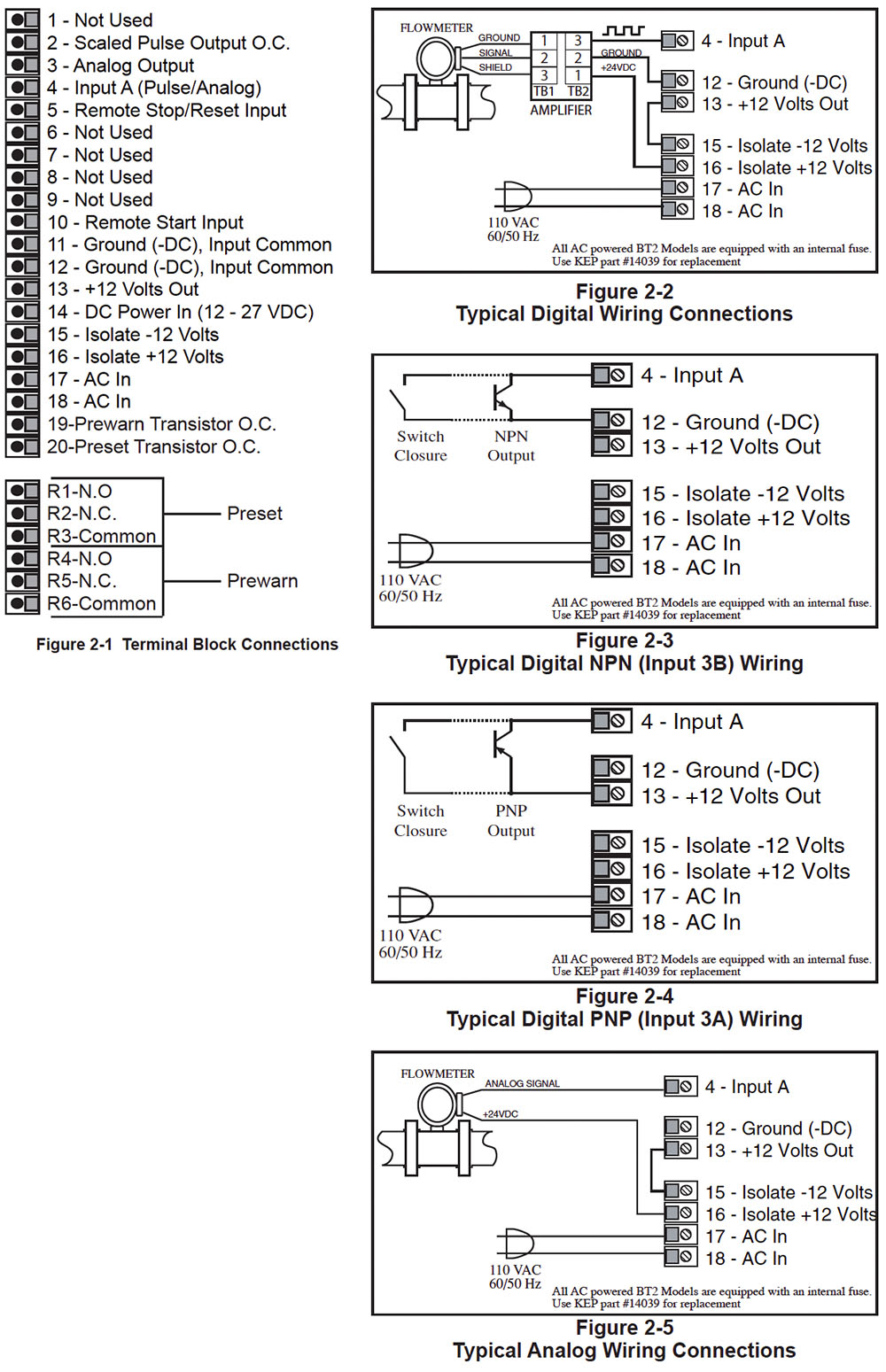# BATCHtrol II (BT2)

Batch Controller with Telephone Type Keypad

Category:

The BATCHtrol II Batch Controller features 8 digits of bright, 0.55 inch, LED display and can accept up to 20 kHz flow input from pulse producing flowmeters. The analog input versions accept inputs, such as 4 to 20 mA or 1 to 5V. The standard unit has two separate, 8 digit, floating decimal, “K” factors to convert the inputs to meaningful total and rate data. An optional 16 point K-factor can linearize flow from nonlinear meter outputs.

## Features

• Easy To Use Batch Controller
• Start/Stop Buttons & Remote Inputs
• Separate 8 Digit K-Factors For Rate & Total
• Displays Rate, Total and Grand Total
• Security Lockout with Missing Pulse Detection
• Scaled Pulse Output
• Two Way RS232/422 Communications Option

(Coming Soon)

## FAQ

For Pulse Inputs the Count K-Factor is equal to the number of pulses per unit of measure which is determined by the flowmeter. (This Information is typically on the flowmeter calibration sheet or stamped on the flowmeter itself).

The Rate K-Factor is calculated by dividing the Count K-Factor by the time conversion factor.

Example:
Count K-Factor = pulses per unit of measure (gallon, foot, revolution etc.…).

Rate K-Factor (rate per second) = pulses per unit / 1
Rate K-Factor (rate per minute) = pulses per unit / 60
Rate K-Factor (rate per hour) = pulses per unit /3600

 Rate K- Factor: 10000/R, where R = high output rating (20mA or 5V) of transmitter. 10000 divided by 20mA or 5V rating of transmitter. Eg. 20mA rating of transmitter is 1500 gal. per min. The rate K – Factor to key into the unit for gal. per min. is 6.6666667 (10000 divided by 1500).The Count K-Factor can be calculated by modifying the Rate K-Factor to reflect the rate in units per second. If the Rate is set for rate per minute, multiply the Rate K-Factor by 60; if rate per hour, multiply the rate K-Factor by 3600.Counter K-Factor: = 6.6666667 x 60 = 400

The Factory back door code for the BATCHtrol II can be obtained by calling the factory or send an email.

Terminal 5 (Stop/Reset Input) has two functions. When activated during a batch, the unit will STOP. When the unit is STOPPED or after a batch is complete, activating this input will reset the Batch Total. If terminal 5 is held high (4-30Vdc), all start inputs will be inhibited. Wiring a push button switch between Terminal 13 and Terminal 5 will accomplish these functions.

 Preset and Prewarn activate the Preset Relay and the Prewarn Relay at a given Batch amount. When entering a value into either one of these setpoints it will cause the corresponding relay to change state at that batch amount.When the start button is pushed, the two relays engage simultaneously to start flow. When the prewarn number is reached, one relay drops out. When the preset number is reached, the other relay drops out. The user may enter the two numbers when setting up the batch counter. The prewarn is set a certain number of counts less than the preset number. If the prewarn is set larger than the preset, the message “PREWRONG” will appear on the disp
 Yes, the BATCHtrol II front panel is NEMA 4X rated. This rating is also comparable to the European standard IP65.

The user can view the Rate, Total and Preset Number by toggling between the three displays with the Rate/Total button on the front of the unit. The user can also view the Grand Total by pushing the “Enter” button once while viewing the Total. The Grand Total will be the flashing Totalizer. Pushing the “Enter” button again will return you to the Total

The Batchtrol II has EEPROM memory and is capable of storing program and count data for a minimum of 10 years. This is also referred to as non-volatile memory

There are two DC outputs available when the unit is powered with AC Voltage. The D.C. outputs are rated at 100ma maximum current drawBelow you will find typical wiring diagrams to help you wire a flowmeter to the BATCHtroll IINumber of items found